CGAL 5.5.2 - Triangulated Surface Mesh Segmentation
User Manual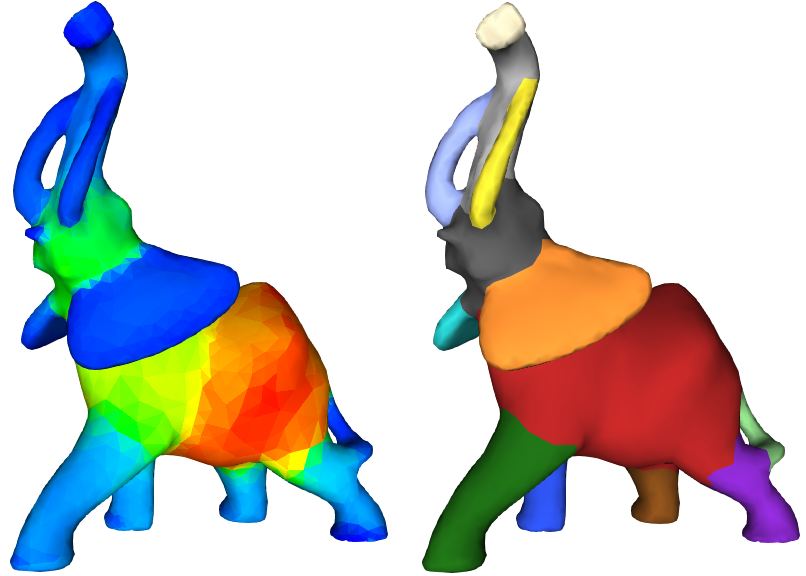Figure 69.1 The shape diameter function applied to the elephant and the corresponding segmentation (using 6 clusters).

# Introduction

Mesh segmentation is the process of decomposing a mesh into smaller and meaningful sub-meshes. This process is used in applications such as modeling, rigging, texturing, shape-retrieval, deformation ... We refer to a comprehensive survey on mesh segmentation  for different segmentation techniques.

This package provides an implementation of the algorithm relying on the Shape Diameter Function  (SDF). Given a triangulated surface mesh (simply mesh in the following) bounding a 3D solid object, the SDF provides an estimate of the local object diameter for each facet of the mesh (the SDF values). The segmentation algorithm first applies a soft clustering on the facets using the associated SDF values. The final segmentation is then obtained via a graph-cut algorithm that considers surface-based features (dihedral-angle and concavity) together with the result of the soft clustering.

This package offers the computation of the SDF values and the mesh segmentation result as independent functions. This allows an alternative implementation of the SDF to be directly plugged into the segmentation algorithm, and also to reuse the SDF values several times with different parameters for the segmentation algorithm.

# Overview of the Segmentation Process

## Shape Diameter Function

The Shape Diameter Function provides a connection between the surface mesh and the volume of the subtended 3D bounded object. More specifically, the SDF is a scalar-valued function defined on facets of the mesh that measures the corresponding local object diameter. The SDF is used to distinguish between thin and thick parts by adding a local notion of thickness to the facets. In addition, the SDF is pose-invariant: SDF values remain largely unaffected after changes of pose (see Figure 69.2).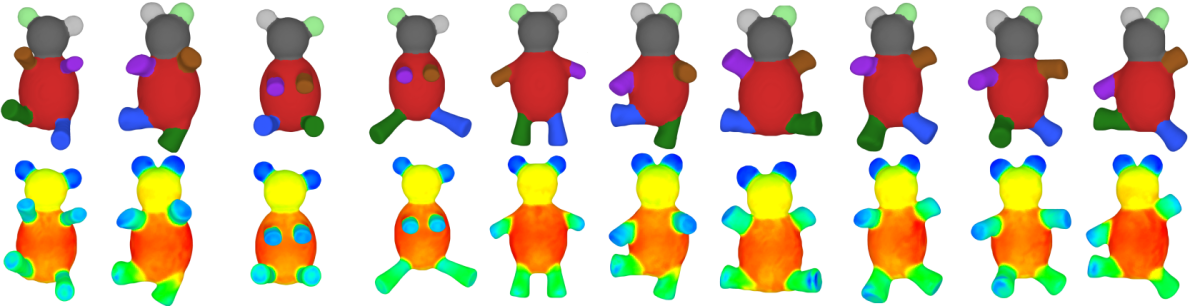Figure 69.2 Influence of pose changes over SDF values and the segmentation.

### Computing Raw SDF Values

For a given input mesh, the raw SDF values are computed by processing each facet one by one. For each facet, several rays are sampled in a cone constructed using the centroid of the facet as apex and inward-normal of the facet as axis. Each ray is truncated into a segment, its endpoints being the apex of the cone and the first mesh facet intersection point. Using the lengths of these truncated rays, which intuitively correspond to a local volume sampling, the raw SDF value is computed by first applying an outlier removal procedure and then taking the average of the lengths.

The raw SDF values are computed through function sdf_values(), setting postprocess to false.

Note
This package also accepts input meshes with holes. In such a case, rays that can not be truncated to segments, or rays that form an obtuse angle with the inward-normal of the first intersected facet are ignored. Only facets having no ray get no raw SDF values

### Post-processing of Raw SDF Values

After having calculated the raw SDF value for each facet, the SDF values used in the segmentation algorithm are the result of several post-processing steps:

• Facets with no raw SDF values are assigned the average raw SDF value of their edge-adjacent neighbors. If there is still a facet having no SDF value, the minimum amongst all the SDF values is assigned to it (this is a deviation from the description of the algorithm in ). The main reason for not assigning 0 to facets with no SDF values is that it can obstruct log-normalization process done at the beginning of segmentation_from_sdf_values().
• A bilateral smoothing  is applied. This smoothing technique removes the noise while trying to keep fast changes on SDF values unchanged since they are natural candidates for segment boundaries. The bilateral smoothing  has three parameters that are set as follows:

• $$w = \lfloor\sqrt{ |F| / 2000}\rfloor + 1$$, the window size (i.e. maximum level for breadth-first neighbor selection), where $$F$$ denotes the set of facets
• $$\sigma_s = w / 2$$, the spatial parameter
• $$\sigma_{r_i} = \sqrt{1/|w_i| \sum_{f_j \in w_i}(SDF(f_j) - SDF(f_i))^2}$$, the range parameter set for each facet $$f_i$$; $$w_i$$ denotes the set of neighboring facets of $$f_i$$ collected using $$w$$ in the facet neighbor breadth-first search

Large window sizes are more effective at eliminating noise but may over-smooth SDF values along segment boundaries. Large range parameters make smoothing closer to Gaussian smoothing and may also lead to over-smoothed SDF values.

• SDF values are linearly normalized between [0,1].

These post-processing steps can be applied to raw SDF values (or an alternative set of similar scalar values associated with facets) using the function sdf_values_postprocessing().

## Soft Clustering

Given a number $$k$$ of clusters, the soft clustering is a Gaussian mixture model that consists in fitting $$k$$ Gaussian distributions to the distribution of the SDF values of the facets. It is initialized with k-means++ , and run multiple times with random seeds. Among these runs, the best result is used for initializing the expectation-maximization algorithm for fitting the Gaussian distributions.

Note
There is no direct relationship between the number of clusters (parameter for soft clustering) and the final number of segments. Intuitively, the number of clusters represents the number of levels of a segmentation by clustering facets that have close SDF values without considering their connectivity. However, a large number of clusters is likely to result in detailed segmentation of the mesh with a large number of segments, see Figure 69.3.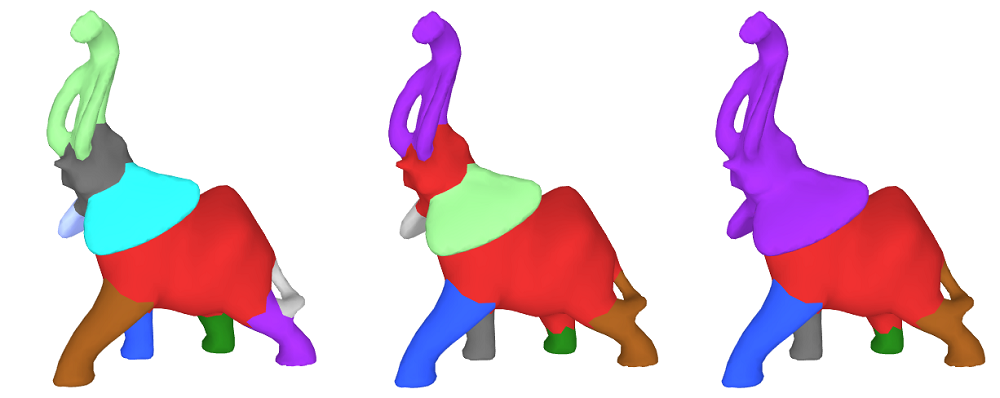Figure 69.3 Influence of the number of clusters over the segmentation. The number of clusters are set to 4, 3, and 2 respectively.

The output of this procedure is a matrix that contains probability values for each facet to belong to each cluster. These probability values are used as input to the hard clustering.

## Hard clustering

The hard clustering yields the final segmentation of the input mesh and results from minimizing an energy function combining the aforementioned probability matrix and geometric surface features.

The energy function minimized using alpha-expansion graph cut algorithm  is defined as follows:

 $$E(\bar{x}) = \sum\limits_{f \in F} e_1(f, x_f) + \lambda \sum\limits_{ \{f,g\} \in N} e_2(x_f, x_g)$$ $$e_1(f, x_f) = -log(max(P(f|x_f), \epsilon))$$ $$e_2(x_f, x_g) = \left \{ \begin{array}{rl} -log(\theta(f,g)/\pi) &\mbox{ x_f \ne x_g} \\ 0 &\mbox{ x_f = x_g} \end{array} \right \}$$ where: $$F$$ denotes the set of facets, $$N$$ denotes the set of pairs of neighboring facets, $$x_f$$ denotes the cluster assigned to facet $$f$$, $$P(f|x_p)$$ denotes the probability of assigning facet $$f$$ to cluster $$x_p$$, $$\theta(f,g)$$ denotes the dihedral angle between neighboring facets $$f$$ and $$g$$: concave angles and convex angles are weighted by 1 and 0.1 respectively, $$\epsilon$$ denotes the minimal probability threshold, $$\lambda \in [0,1]$$ denotes a smoothness parameter.

The first term of the energy function provides the contribution of the soft clustering probabilities. The second term of the energy function is a geometric criterion that is larger when two adjacent facets sharing a sharp and concave edge are in the same cluster. The smoothness parameter makes this geometric criteria more or less prevalent.

Assigning a high value to the smoothness parameter results in a small number of segments (since constructing a segment boundary would be expensive). In other words, merging facets that are placed under different clusters is less expensive than separating them and creating boundaries. On the contrary, assigning smaller values to smoothness parameter results in a high number of segments, by getting closer to the result of the soft clustering (notice that setting $$\lambda=0$$ provides the result of the soft clustering). Figure 69.4 depicts the influence of the smoothness parameter.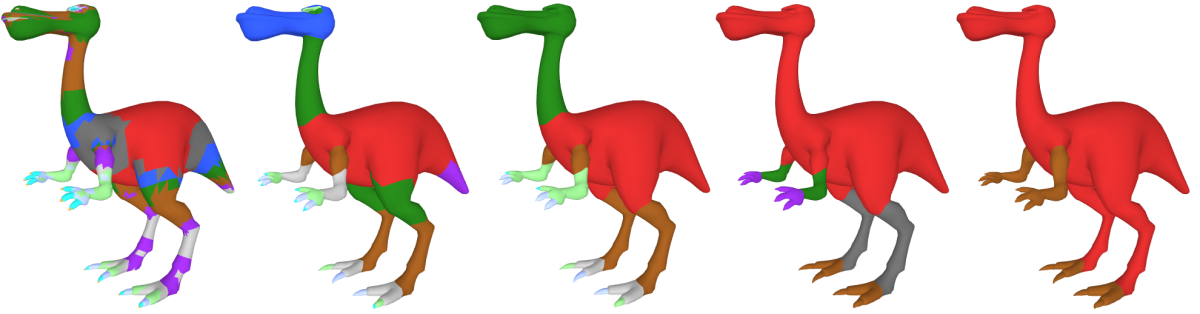Figure 69.4 Influence of the smoothness parameter lambda over the segmentation (using 10 clusters). Smoothness parameters are set to 0.0, 0.1, 0.25, 0.5 and 1.0 respectively. The coloring reflects the segmentation result before assigning each connected component to its own segment.

The hard clustering assigns a cluster id to each facet (see Figure 69.5 (a)). A segment consists in a set of connected facets in the same cluster (see Figure 69.5 (b)). By default the function segmentation_from_sdf_values() assigns to each facet the id of its segment. It assigns to each facet the id of its cluster when output_cluster_ids is set to true.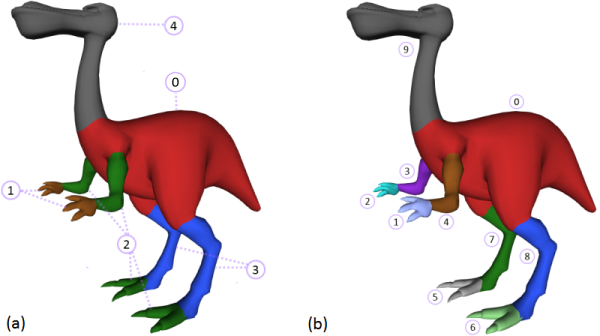Figure 69.5 Clusters and segments. The input number of clusters is set to 5. (a) Result of the hard-clustering. (b) Segments are extracted from the computed clusters.

## Summary

Four functions are provided:

• sdf_values() : computes the SDF value of each facet of an input mesh in either raw or post-processed form. SDF values are associated to facets using a property map (see CGAL and Boost Property Maps).
• sdf_values_postprocessing() : post-processes raw SDF values. The post-processing is decoupled from the function sdf_values() to allow the use of alternative methods to compute SDF values or additional post-processing step.
• segmentation_from_sdf_values() : computes the mesh segmentation from the SDF values of the facets of an input mesh. The input SDF values can be any set of scalar values associated to each facet as long as they have been normalized between 0 and 1. This function allows using the same SDF values with different parameters for the segmentation stage. The segment or cluster ids are associated to the facets using a property map.
• segmentation_via_sdf_values() : combines the three functions above.

These functions expect as input a triangulated surface mesh bounding a 3D solid object, with the following properties:

• Combinatorially 2-manifold;
• The vertices of the facets are oriented counterclockwise when seen from outside of the object;
• Intersection-free;
• Boundary free.
Note
The current implementation executes fine on meshes that do not match some of these properties but may produce unreliable or meaningless segmentations.

The current implementation of the computation of the SDF values relies on the 3D Fast Intersection and Distance Computation package. This operation is reliable when the AABBTraits model provided has exact predicates. CGAL::Exact_predicates_inexact_constructions_kernel is recommended as geometric traits for this algorithm.

# Examples

## Example: Computation of SDF Values

#include <CGAL/Exact_predicates_inexact_constructions_kernel.h>
#include <CGAL/Polyhedron_3.h>
#include <CGAL/mesh_segmentation.h>
#include <CGAL/property_map.h>
#include <iostream>
#include <fstream>
typedef CGAL::Polyhedron_3<Kernel> Polyhedron;
typedef boost::graph_traits<Polyhedron>::face_descriptor face_descriptor;
int main()
{
Polyhedron mesh;
std::ifstream input(CGAL::data_file_path("meshes/cactus.off"));
if ( !input || !(input >> mesh) || mesh.empty() || ( !CGAL::is_triangle_mesh(mesh)) )
{
std::cerr << "Input is not a triangle mesh" << std::endl;
return EXIT_FAILURE;
}
// create a property-map
typedef std::map<face_descriptor, double> Face_double_map;
Face_double_map internal_map;
boost::associative_property_map<Face_double_map> sdf_property_map(internal_map);
// compute SDF values
std::pair<double, double> min_max_sdf = CGAL::sdf_values(mesh, sdf_property_map);
// It is possible to compute the raw SDF values and post-process them using
// the following lines:
// const std::size_t number_of_rays = 25; // cast 25 rays per face
// const double cone_angle = 2.0 / 3.0 * CGAL_PI; // set cone opening-angle
// CGAL::sdf_values(mesh, sdf_property_map, cone_angle, number_of_rays, false);
// std::pair<double, double> min_max_sdf =
// CGAL::sdf_values_postprocessing(mesh, sdf_property_map);
// print minimum & maximum SDF values
std::cout << "minimum SDF: " << min_max_sdf.first
<< " maximum SDF: " << min_max_sdf.second << std::endl;
// print SDF values
for(face_descriptor f : faces(mesh))
std::cout << sdf_property_map[f] << " ";
std::cout << std::endl;
return EXIT_SUCCESS;
}

## Example: Segmentation from SDF Values

#include <CGAL/Exact_predicates_inexact_constructions_kernel.h>
#include <CGAL/Polyhedron_3.h>
#include <CGAL/mesh_segmentation.h>
#include <CGAL/property_map.h>
#include <iostream>
#include <fstream>
typedef CGAL::Polyhedron_3<Kernel> Polyhedron;
typedef boost::graph_traits<Polyhedron>::face_descriptor face_descriptor;
int main()
{
Polyhedron mesh;
std::ifstream input(CGAL::data_file_path("meshes/cactus.off"));
if ( !input || !(input >> mesh) || mesh.empty() || ( !CGAL::is_triangle_mesh(mesh)))
{
std::cerr << "Input is not a triangle mesh." << std::endl;
return EXIT_FAILURE;
}
// create a property-map for SDF values
typedef std::map<face_descriptor, double> Facet_double_map;
Facet_double_map internal_sdf_map;
boost::associative_property_map<Facet_double_map> sdf_property_map(internal_sdf_map);
// compute SDF values using default parameters for number of rays, and cone angle
CGAL::sdf_values(mesh, sdf_property_map);
// create a property-map for segment-ids
typedef std::map<face_descriptor, std::size_t> Facet_int_map;
Facet_int_map internal_segment_map;
boost::associative_property_map<Facet_int_map> segment_property_map(internal_segment_map);
// segment the mesh using default parameters for number of levels, and smoothing lambda
// Any other scalar values can be used instead of using SDF values computed using the CGAL function
std::size_t number_of_segments = CGAL::segmentation_from_sdf_values(mesh, sdf_property_map, segment_property_map);
std::cout << "Number of segments: " << number_of_segments << std::endl;
// print segment-ids
for(face_descriptor f : faces(mesh)) {
// ids are between [0, number_of_segments -1]
std::cout << segment_property_map[f] << " ";
}
std::cout << std::endl;
const std::size_t number_of_clusters = 4; // use 4 clusters in soft clustering
const double smoothing_lambda = 0.3; // importance of surface features, suggested to be in-between [0,1]
// Note that we can use the same SDF values (sdf_property_map) over and over again for segmentation.
// This feature is relevant for segmenting the mesh several times with different parameters.
mesh, sdf_property_map, segment_property_map, number_of_clusters, smoothing_lambda);
return EXIT_SUCCESS;
}

### Computation of SDF Values and Segmentation

The function segmentation_via_sdf_values() combines the computation of sdf values, the post-processing and the segmentation. Note that when computing several segmentations of a mesh with different parameters (i.e. number of levels, and smoothing lambda), it is advised to first compute the SDF values using sdf_values() and use them in calls of the function segmentation_from_sdf_values().

#include <CGAL/Exact_predicates_inexact_constructions_kernel.h>
#include <CGAL/Polyhedron_3.h>
#include <CGAL/mesh_segmentation.h>
#include <CGAL/property_map.h>
#include <iostream>
#include <fstream>
typedef CGAL::Polyhedron_3<Kernel> Polyhedron;
typedef boost::graph_traits<Polyhedron>::face_descriptor face_descriptor;
int main()
{
Polyhedron mesh;
std::ifstream input(CGAL::data_file_path("meshes/cactus.off"));
if ( !input || !(input >> mesh) || mesh.empty() || ( !CGAL::is_triangle_mesh(mesh)) )
{
std::cerr << "Input is not a triangle mesh" << std::endl;
return EXIT_FAILURE;
}
// create a property-map for segment-ids
typedef std::map<face_descriptor, std::size_t> Face_int_map;
Face_int_map internal_segment_map;
boost::associative_property_map<Face_int_map> segment_property_map(internal_segment_map);
// calculate SDF values and segment the mesh using default parameters.
std::size_t number_of_segments = CGAL::segmentation_via_sdf_values(mesh, segment_property_map);
std::cout << "Number of segments: " << number_of_segments << std::endl;
// print segment-ids
for(face_descriptor f : faces(mesh) ) {
std::cout << segment_property_map[f] << " ";
}
std::cout << std::endl;
return EXIT_SUCCESS;
}

## Using a Polyhedron with an ID per Facet

The previous examples use a std::map as property maps for storing the SDF values and the segmentation results. This example uses a polyhedron type with a facet type storing an extra ID field, together with a vector, as underlying data structure in the property maps. The main advantage is to decrease from log to constant the complexity for accessing the data associated to facets.

#include <CGAL/Exact_predicates_inexact_constructions_kernel.h>
#include <CGAL/Polyhedron_items_with_id_3.h>
#include <CGAL/Polyhedron_3.h>
#include <CGAL/mesh_segmentation.h>
#include <CGAL/property_map.h>
#include <iostream>
#include <fstream>
typedef CGAL::Polyhedron_3<K, CGAL::Polyhedron_items_with_id_3> Polyhedron;
typedef boost::graph_traits<Polyhedron>::face_descriptor face_descriptor;
// Property map associating a face with an integer as id to an
// element in a vector stored internally
template<class ValueType>
struct Face_with_id_pmap
: public boost::put_get_helper<ValueType&,
Face_with_id_pmap<ValueType> >
{
typedef face_descriptor key_type;
typedef ValueType value_type;
typedef value_type& reference;
typedef boost::lvalue_property_map_tag category;
Face_with_id_pmap(
std::vector<ValueType>& internal_vector
) : internal_vector(internal_vector) { }
reference operator[](key_type key) const
{ return internal_vector[key->id()]; }
private:
std::vector<ValueType>& internal_vector;
};
int main()
{
Polyhedron mesh;
std::ifstream input(CGAL::data_file_path("meshes/cactus.off"));
if ( !input || !(input >> mesh) || mesh.empty() || ( !CGAL::is_triangle_mesh(mesh)) ) {
std::cerr << "Input is not a triangle mesh" << std::endl;
return EXIT_FAILURE;
}
// assign id field for each face
std::size_t face_id = 0;
for(face_descriptor f : faces( mesh) ) {
f->id() = face_id++;
}
// create a property-map for SDF values
std::vector<double> sdf_values(num_faces(mesh));
Face_with_id_pmap<double> sdf_property_map(sdf_values);
CGAL::sdf_values(mesh, sdf_property_map);
// access SDF values (with constant-complexity)
for(face_descriptor f : faces(mesh)) {
std::cout << sdf_property_map[f] << " ";
}
std::cout << std::endl;
// create a property-map for segment-ids
std::vector<std::size_t> segment_ids(num_faces(mesh));
Face_with_id_pmap<std::size_t> segment_property_map(segment_ids);
CGAL::segmentation_from_sdf_values(mesh, sdf_property_map, segment_property_map);
// access segment-ids (with constant-complexity)
for(face_descriptor f : faces(mesh)) {
std::cout << segment_property_map[f] << " ";
}
std::cout << std::endl;
return EXIT_SUCCESS;
}

## Using a Surface_mesh

When using a Surface_mesh, you can use the built-in property mechanism.

#include <CGAL/Exact_predicates_inexact_constructions_kernel.h>
#include <CGAL/Surface_mesh.h>
#include <CGAL/mesh_segmentation.h>
#include <CGAL/Polygon_mesh_processing/IO/polygon_mesh_io.h>
#include <CGAL/property_map.h>
#include <iostream>
#include <fstream>
typedef Kernel::Point_3 Point_3;
typedef boost::graph_traits<Mesh>::vertex_descriptor vertex_descriptor;
typedef boost::graph_traits<Mesh>::face_descriptor face_descriptor;
int main(int argc, char** argv )
{
const std::string filename = (argc > 1) ? argv : CGAL::data_file_path("meshes/cactus.off");
Mesh mesh;
{
std::cerr << "Invalid input file." << std::endl;
return EXIT_FAILURE;
}
typedef Mesh::Property_map<face_descriptor,double> Facet_double_map;
Facet_double_map sdf_property_map;
// compute SDF values
// We can't use default parameters for number of rays, and cone angle
// and the postprocessing
CGAL::sdf_values(mesh, sdf_property_map, 2.0 / 3.0 * CGAL_PI, 25, true);
// create a property-map for segment-ids
typedef Mesh::Property_map<face_descriptor, std::size_t> Facet_int_map;
// segment the mesh using default parameters for number of levels, and smoothing lambda
// Any other scalar values can be used instead of using SDF values computed using the CGAL function
std::size_t number_of_segments = CGAL::segmentation_from_sdf_values(mesh, sdf_property_map, segment_property_map);
std::cout << "Number of segments: " << number_of_segments << std::endl;
// print segment-ids
for(face_descriptor fd : faces(mesh)){
// ids are between [0, number_of_segments -1]
std::cout << segment_property_map[fd] << " ";
}
std::cout << std::endl;
const std::size_t number_of_clusters = 4; // use 4 clusters in soft clustering
const double smoothing_lambda = 0.3; // importance of surface features, suggested to be in-between [0,1]
// Note that we can use the same SDF values (sdf_property_map) over and over again for segmentation.
// This feature is relevant for segmenting the mesh several times with different parameters.
CGAL::segmentation_from_sdf_values(mesh, sdf_property_map, segment_property_map, number_of_clusters, smoothing_lambda);
return EXIT_SUCCESS;
}

## Independent TriangleMesh per Segment

It is possible to consider each segment as an independent triangle mesh, like in the following example, where the area of each segment is computed.

#include <CGAL/Exact_predicates_inexact_constructions_kernel.h>
#include <CGAL/Surface_mesh.h>
#include <CGAL/boost/graph/copy_face_graph.h>
#include <CGAL/boost/graph/Face_filtered_graph.h>
#include <CGAL/Polygon_mesh_processing/measure.h>
#include <CGAL/Polygon_mesh_processing/IO/polygon_mesh_io.h>
#include <CGAL/mesh_segmentation.h>
#include <iostream>
#include <fstream>
#include <sstream>
typedef boost::graph_traits<SM>::face_descriptor face_descriptor;
int main(int argc, char** argv )
{
const std::string filename = (argc > 1) ? argv : CGAL::data_file_path("meshes/cactus.off");
SM mesh;
{
std::cerr << "Invalid input file." << std::endl;
return EXIT_FAILURE;
}
typedef SM::Property_map<face_descriptor,double> Facet_double_map;
Facet_double_map sdf_property_map;
CGAL::sdf_values(mesh, sdf_property_map);
// create a property-map for segment-ids
typedef SM::Property_map<face_descriptor, std::size_t> Facet_int_map;
// segment the mesh using default parameters for number of levels, and smoothing lambda
// Any other scalar values can be used instead of using SDF values computed using the CGAL function
std::size_t number_of_segments = CGAL::segmentation_from_sdf_values(mesh, sdf_property_map, segment_property_map);
typedef CGAL::Face_filtered_graph<SM> Filtered_graph;
//print area of each segment and then put it in a Mesh and print it in an OFF file
Filtered_graph segment_mesh(mesh);
for(std::size_t id = 0; id < number_of_segments; ++id)
{
segment_mesh.set_selected_faces(id, segment_property_map);
std::cout << "Segment "<<id<<"'s area is : "<<CGAL::Polygon_mesh_processing::area(segment_mesh)<<std::endl;
SM out;
CGAL::copy_face_graph(segment_mesh, out);
std::ostringstream oss;
oss << "Segment_" << id<<".off";
std::ofstream os(oss.str().data());
os<<out;
}
}

# Performances

The following tables provide the runtime of the functions sdf_values() and segmentation_from_sdf_values(). The results were produced with the release 4.4 of CGAL, on an Intel i7 3.2 Ghz laptop with 8 GB RAM, compiled by Visual C++ 2010 with /O2 option. The polyhedron types are using Polyhedron_items_with_id_3 as item class. The models used for the benchmarks are the dinosaur model with 7,828 facets, the bear model with 20,188 facets and the elephant model with 88,928 facets.

Runtime in seconds of sdf_values() with 25 rays showing the cost of the robustness:

Number of triangles Simple_cartesian<double> Exact_predicates_inexact_constructions_kernel (EPICK)
7,828 2.3 3.8
20,188 6.1 9.5
88,928 46.1 62.3

Runtime in milliseconds of segmentation_from_sdf_values() (using Simple_cartesian<double> or EPICK gives the same results), the graph-cut part is using the library MaxFlow v2.21:

Number of triangles Number of cluster = 2 Number of cluster = 5 Number of cluster = 10 Number of cluster = 15
7,828 38 61 141 204
20,188 50 163 483 608
88,928 314 1,260 2,736 4,239

# Design and Implementation History

The initial implementation of this package is the result of the work of Ilker O. Yaz during the 2012 season of the Google Summer of Code. He has been mentored by Sébastien Loriot who also contributed to the documentation and the API definition.